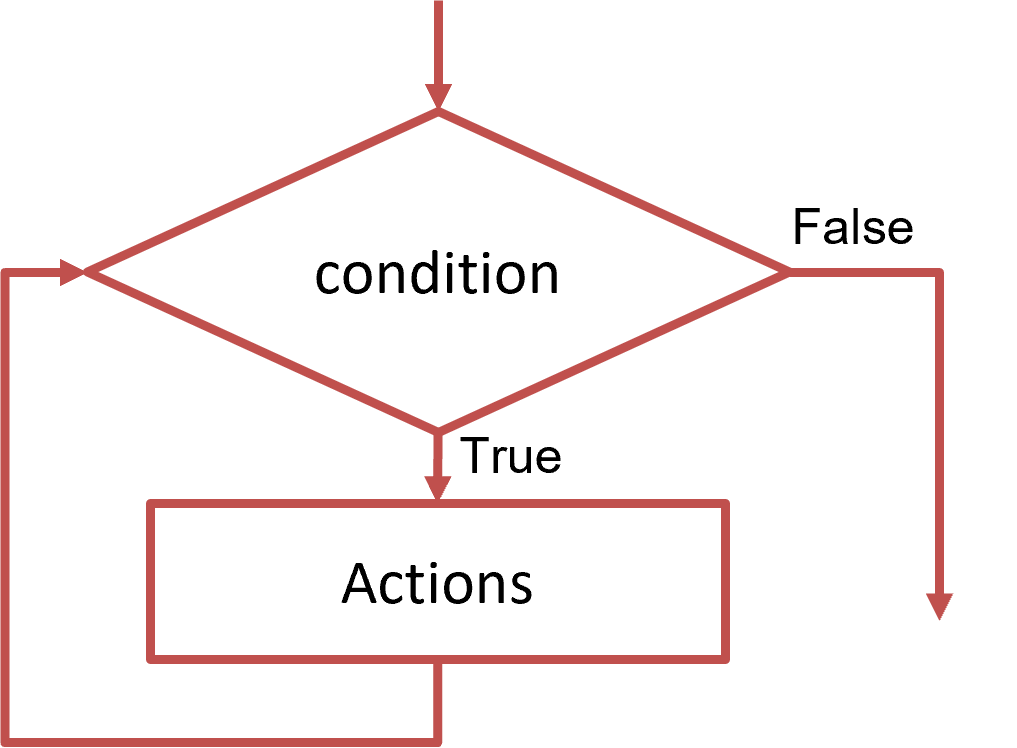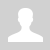Python. WHILE Loop

11.1.2.1 write program code using a While loop

Python. WHILE Loop

### Why do we need loops?

We have already learned how to check a condition in a program. But what if certain actions are repeated several times? Of course, you can write program code for each of these actions. But if these actions are repeated dozens, hundreds, thousands, millions of times, then our program will be very long.

To repeat actions in the program several times, we use the loops.

A loop is an algorithm structure that executes a sequence of instructions multiple times.

### WHILE Loop

We will first explore the use of the while loop. The while statement ("bye") checks the condition and performs some actions (the body of the loop ) while the loop condition is true.

A preconditioned loop has the following structure:``````while [condition]: # checking the loop condition
action1 # loop body (actions that are repeated)
action2 # is executed WHILE the condition is met
... # each line in the body of the loop is indented - 4 spaces
actionN``````

To make it easier to understand the program, the entire body of the loop and its bounding parentheses are shifted to the right.

There are a few more important concepts to know:

1. The body of the loop is the sequence of code that needs to be executed several times.
2. One-time execution is iteration.

Features of the while loop:

• The while loop is used when the number of loop repetitions is not known in advance and cannot be calculated.
• The while loop consists of a head and a loop body.
• In the heading, after the word while, a condition is written under which the loop continues to run in parentheses. When this condition is violated (becomes false), the cycle ends.
• In this condition, you can use the signs of logical relations and operations, as in the Conditional operator.
• If the condition is incorrect initially, then the loop will not be executed even once.
• If the condition never becomes false (false), then the loop will never end; in this case, they say that the program is "infinite looped ").
• In the C language, any number that is not equal to zero denotes a true condition, and zero denotes a false word:
``````while True: # starts an infinite loop
...
while False: # the loop will not be executed even once
...``````

Task 1 . Write a program that asks for a password until "qwerty" is entered.

It is often, impossible to say in advance how many times an operation needs to be performed, but it is possible to determine the condition under which it should end.

In this program, the user can enter the password incorrectly; then, the program will report an error and ask for it again until the correct password is entered.

To solve this problem, we must use a loop condition to validate the password after each input. For this, the password will be entered at the beginning of the program and inside the loop.

```print("Enter password:") password = input () # enter password, set the first value while password != "qwerty": # check the condition of the loop     print ("The password is incorrect!")     print ("Enter password:")     password = input () # re-enter password print ("Welcome!") # output text when entering password "qwerty"```

Consider another use of the while loop.

Task 2. Calculate the sum of the sequence 1 + 3 + 5 + ... + n

You can use a loop to calculate the amount. In this sequence, you can notice that each next term is increased by 2. Let us denote the term by the variable i and will change it in the loop. The initial value of the variable i is 1, the final value is n.

To calculate the amount, we will use the formulas:

```sum += i i += 2```

```summa = 0 # initial value of the sum i = 1 # initial value of the loop parameter n = int(input ()) # input of the final value of the loop parameter while i <= n: # loop condition "while i <= n"     summa += i # increase the sum by i     i += 2 # increase the loop parameter by 2 print(summa) # output the value of the sum```

Questions:

Exercises:

Категория: Flowcharts | Добавил: bzfar77 (27.09.2021)
Просмотров: 468 | Теги: Loop, While, Iteration, Python | Рейтинг: 0.0/0
 Всего комментариев: 0